Painlevé test

Painlevé analysis

Suppose one reduces a partial differential equation to an ordinary differential equation by (similarity) reduction. The question arises as to whether the ordinary differential equation possesses movable critical points (cf. Movable singular point). If the equation happens to be of second order, one can consult [a1]. If one can transform the equation into one of the 50 canonical types, then the equation has the Painlevé property (cf. Painlevé-type equations). If the equation cannot be transformed to one of those on the list, then it is not of Painlevé type. If the equation is of third or higher order, a procedure described in [a3], [a4] determines if the neighbourhood of an algebraic singularity will be single valued (i.e. if it possesses a Laurent series). The method is similar to that proposed by S. Kovalevskaya (cf. Cauchy–Kovalevskaya theorem). A typical calculation runs as follows.

Generalized cylindrical Korteweg–de Vries equations (KdV, cf. Korteweg–de Vries equation) are of the formwhereis an arbitrary constant. This equation admits a similarity solution: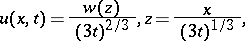where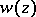satisfies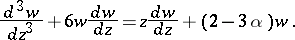It is easy to verify that the equation has a movable algebraic singularity with the following expansion: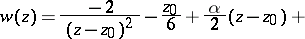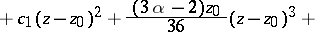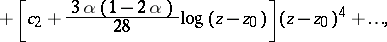whereare the three arbitrary constants necessary for a "full-dimensional family" of solutions of the above third-order ordinary differential equation. It is clear that the solution possesses a movable (logarithmic) branch point (cf. Branch point) unless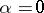,. In the latter cases the expansion can be continued to arbitrarily high order and, in fact, both cases(KdV)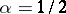(the cylindrical KdV equation) are known to be integrable. The Painlevé test is used as a means to predict whether an equation is likely to be integrable. There are numerous examples of the success of the method. See [a3], [a4] for other examples and references.

A drawback to applying the above test to a given partial differential equation is the need to reduce the equation by (similarity) reduction to an ordinary differential equation. Sometimes there no reductions possible or the reductions are trivial. In [a5] (some other examples are discussed in [a4]) an extension of the above Painlevé test to partial differential equations was developed. It is very similar in spirit to the tests developed for ordinary differential equations in the sense that one looks for Laurent-type series (as a function of several complex variables). The method has been used successfully in many examples.

There has been considerable research in Painlevé-type equations from the 1980s onwards. Despite the fact that the analysis and studies are not fully comprehensive (i.e. they do not cover all possible singular points) and although at times it is purely formal (tests for partial differential equations), nevertheless it is undeniable that "in practice" the Painlevé tests have been remarkable in their ability to be able to predict whether an equation is integrable. The main issue is this. If a partial or ordinary differential equation has a sufficiently "simple" complex structure, i.e. if all its solutions are single valued or transformable to such, then it is integrable in the sense of complex variables. Its solutions can be eventually continued and connected throughout the complex plane. It is remarkable that many fundamentally important equations that arise in physical applications have such a simple complex structure.